# Solving Systems of Linear and Quadratic Equations Graphically

(also see Systems of Linear and Quadratic Equations)A Linear Equation is an equation of a line.A Quadratic Equation is the equation of a parabola and has at least one variable squared (such as x2)And together they form a System of a Linear and a Quadratic Equation

A System of those two equations can be solved (find where they intersect), either:

• Using Algebra
• Or Graphically, as we will find out!

## How to Solve Graphically

Easy! Plot both equations and see where they cross!

### Plotting the Equations

We can plot them manually, or use a tool like the Function Grapher.

To plot them manually:

• make sure both equations are in "y=" form
• choose some x-values that will hopefully be near where the two equations cross over
• calculate y-values for those x-values
• plot the points and see!

### Choosing Where to Plot

But what values should we plot? Knowing the center will help!

Take the quadratic formula and ignore everything after the ± gets us a central x-value:Then choose some x-values either side and calculate y-values, like this:

### Example: Solve these two equations graphically to 1 decimal place:

• y = x2 − 4x + 5
• y = x + 2

Find a Central X Value:

The quadratic equation is y = x2 − 4x + 5, so a = 1, b = −4 and c = 5

Central x = −b2a = −(−4)2×1 = 42 = 2

Now Calculate Values Around x=2

x
x2 − 4x + 5
Linear
x + 2
0 5 2
1 2
2 1
3 2
4 5
5 10 7

(We only calculate first and last of the linear equation as that is all we need for the plot.)

Now Plot Them: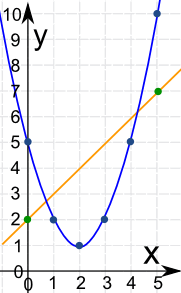We can see they cross at about x = 0.7 and about x = 4.3

Let us do the calculations for those values:

x
x2 − 4x + 5
Linear
x + 2
0.7 2.69 2.8
4.3 6.29 6.2

Yes they are close.

To 1 decimal place the two points are (0.7, 2.8) and (4.3, 6.2)

## There Might Not Be 2 Solutions!

There are three possible cases:

• No real solution (happens when they never intersect)
• One real solution (when the straight line just touches the quadratic)
• Two real solutions (like the example above)Time for another example:

### Example: Solve these two equations graphically:

• 4y − 8x = −40
• y − x2 = −9x + 21

How do we plot these? They are not in "y=" format!

First make both equations into "y=" format:

Linear equation is: 4y − 8x = −40

Add 8x to both sides: 4y = 8x − 40
Divide all by 4: y = 2x − 10

Quadratic equation is: y − x2 = −9x + 21

Add x2 to both sides: y = x2 − 9x + 21

Now Find a Central X Value:

The quadratic equation is y = x2 − 9x + 21, so a = 1, b = −9 and c = 21

Central x = −b2a = −(−9)2×1 = 92 = 4.5

Now Calculate Values Around x=4.5

x
x2 − 9x + 21
Linear
2x − 10
3 3 −4
4 1
4.5 0.75
5 1
6 3
7 7 4

Now Plot Them: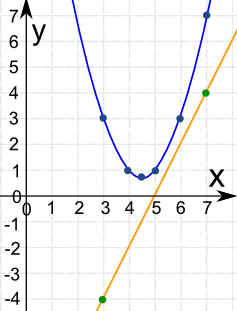They never cross! There is no solution.### Real World Example

Kaboom!

The cannon ball flies through the air, following a parabola:

y = 2 + 0.12x - 0.002x2

The land slopes upward: y = 0.15x

Where does the cannon ball land?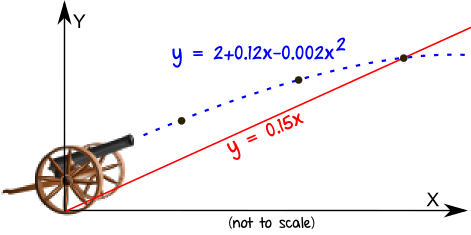Let's fire up the Function Grapher!

Enter 2 + 0.12x - 0.002x^2 for one function and 0.15x for the other.

Zoom out, then zoom in where they cross. You should get something like this: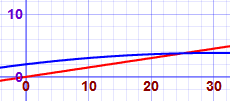By zooming in far enough we can find they cross at (25, 3.75)

## Circle and Line

### Example: Find the points of intersection to 1 decimal place of

• The circle x2 + y2 = 25
• And the straight line 3y - 2x = 6

### The Circle

The "Standard Form" for the equation of a circle is (x-a)2 + (y-b)2 = r2

Where (a, b) is the center of the circle and r is the radius.

For x2 + y2 = 25 we can see that

• a=0 and b=0 so the center is at (0, 0),
• and for the radius r2 = 25 , so r = √25 = 5

We don't need to make the circle equation in "y=" form, as we have enough information to plot the circle now.

### The Line

First put the line in "y=" format:

Move 2x to right hand side: 3y = 2x + 6
Divide by 3: y = 2x/3 + 2

To plot the line, let's choose two points either side of the circle:

• at x = −6, y = (2/3)(6) + 2 = −2
• at x = 6, y = (2/3)(6) + 2 = 6

Now plot them!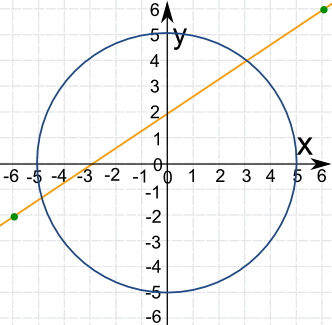We can now see that they cross at about (-4.8, -1.2) and (3.0, 4.0)

For an exact solution see Systems of Linear and Quadratic Equations

8199, 8200, 8201, 8202, 8203, 8204, 8205, 8206, 8207, 8208